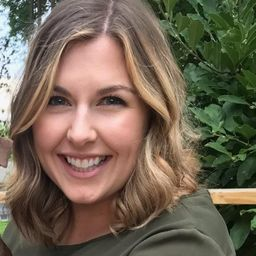Our Discord hit 10K members! 🎉 Meet students and ask top educators your questions.Join Here!Numerade Educator

# The region enclosed by the given curves is rotated about the specified line. Find the volume of the resulting solid.$$y=x^{3}, y=\sqrt{x} ; \quad \text { about } x=1$$

## $=\frac{13 \pi}{30}$

#### Topics

Applications of Integration

### Discussion

You must be signed in to discuss.
##### Top Calculus 2 / BC Educators##### Catherine R.

Missouri State University##### Samuel H.

University of Nottingham##### Michael J.

Idaho State University### Video Transcript

I'm gonna find the volume. And I have these two equations that I am using two bound to the area. So the area bounded by X cubed in the square root of X. Is this part right here? So once I figure out that two dimensional area that I need to see where it's revolving around in this case, let's revolving around the vertical line. X equals one. So if we were evolved this area around that vertical line X equals one. Notice that we have all of this space between what we've shaded in and where were rotating, which means this is a washer set up. So for the washer set up, we use the equals. Volume equals pi times the integral of R squared minus little r squared. And then we want to use whatever variable is the axis. Well, in this case, X equals one is running along like it's the why access. It's parallel to the y axis. So I used d y, which means my limits on the integral, whatever I feel into the ours and that in a girl for variation. Sorry. The variable for integration needs all match. Why? Why? Why? So then we need to find that highest and lowest y value the limits here for integral. So So, Lois, why value I hope it's clear happens at the origin. And then you may realize that the cubic graph and the square root graft me at the 0.11 which means your upper bound for why here is why equals one so filling in your integral. You have high temps in a girl going from 0 to 1. We know that much already. So now you didn't decide what is capital are. And then we have to decide what is lower case art capital are is what if everything was filled in? What if there was no missing volume? Well, based on this shape I have shaded in and where I'm revolving, that would mean I would actually need to color in this entire wedge here. It would have to go all the way up against X equals one, so that would be defined by one minus the square root of X one minus that curbed graph. But this is a problem. I don't want to use the square root of X here because I wanted to be all in terms of why. So we need to rewrite this equation in terms of why? Which means solve it for X equals. So X equals Y squared is actually how I would solve that curve graph. And that means I want to fill in. Why squared into that capital are instead of the square it function? So that's why it's really important for me to think about over here at the start. Hey, this needs to all be in terms of Why so that way don't have that random square root function going through with the axe with the wrong. Very well. So now for lower case R, I want to fill in. What stuff am I taking away? Well, stuff I'm taking away is between the share it shaded area in the axis. So that's going from one until the cubic graph. Well, again, I don't want to use X, so we need to solve that cubic equation to be in terms of why? Well, if I got X by itself, I would have to a cube root the other side so I would actually fill in one minus the cube root of why. And that's gonna be my little are one minus the cube root of why? And I have capital, Art and lower case are building to my set up for the volume, and I'm ready to calculate this integral. So I type in actually just integral portion of this into maybe my online software or my calculator. I get 13/30 and then multiplying by pie. I get 13 pi over 30 and that's my final answer for this volume.Oklahoma Baptist University

#### Topics

Applications of Integration

##### Top Calculus 2 / BC Educators##### Catherine R.

Missouri State University##### Samuel H.

University of Nottingham##### Michael J.

Idaho State University